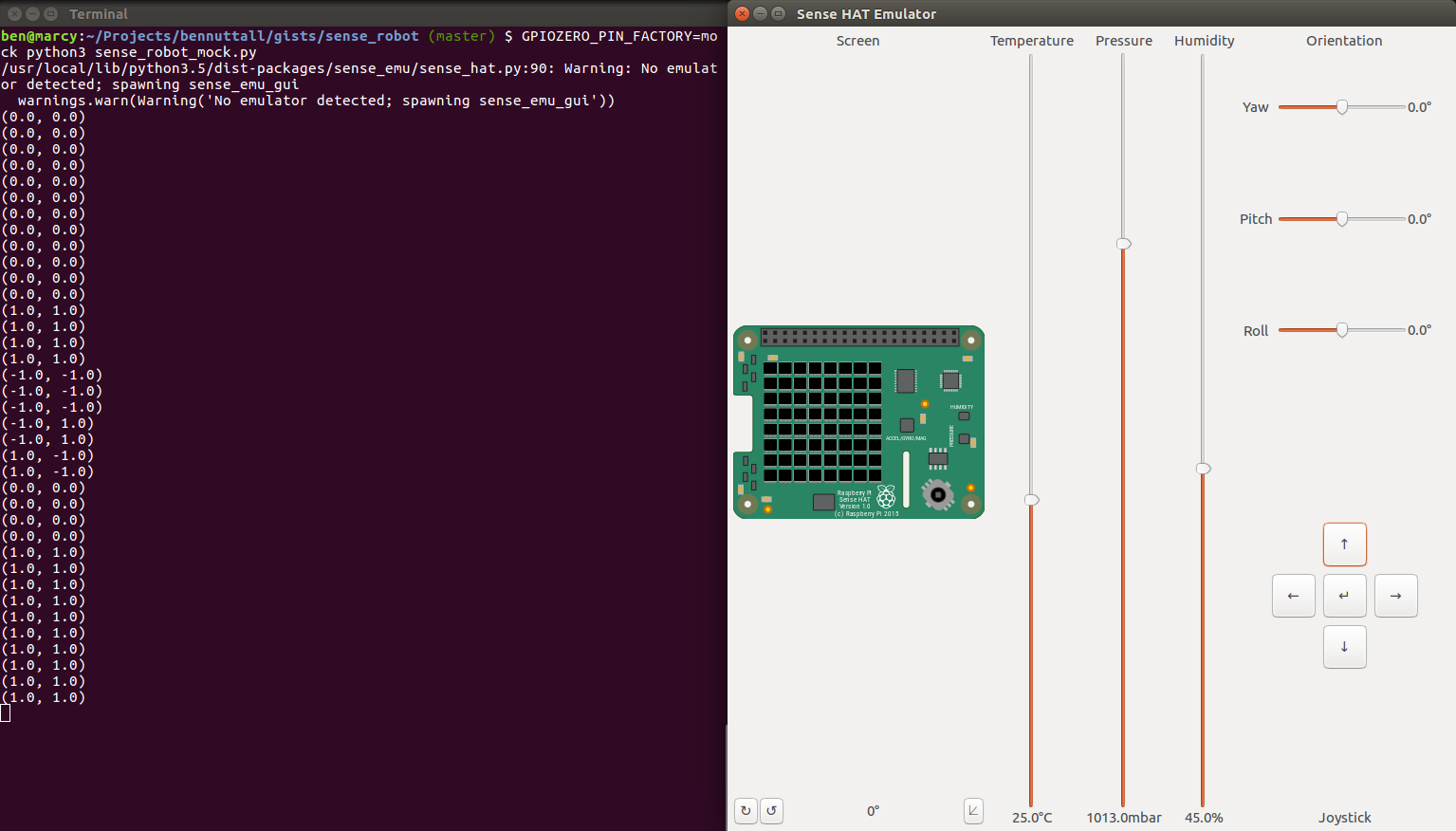## Prototyping a Raspberry Pi robot idea with two emulators

While preparing for a workshop last week, my colleague Marc and I started brainstorming ideas. One of the ideas I came up with was to use the mini joystick on a Sense HAT (a sensor board add-on for the Raspberry Pi) to remotely control a robot using GPIO Zero’s remote pins feature. I soon started writing the code for it on my laptop. Then I realised I could actually prototype the whole idea without touching a Raspberry Pi, using a combination of the Sense HAT’s desktop emulator developed by Dave Jones, and GPIO Zero’s mockpin interface (erm… also developed by Dave Jones).

The first proof-of-concept demo was really simple: it was just a case of connecting the joystick events with robot actions, i.e. joystick up => robot forward, and so on:

```from gpiozero import Robot
from sense_emu import SenseHat
from time import sleep

sense = SenseHat()
robot = Robot(left=(2, 3), right=(4, 5), pwm=False)

sense.stick.direction_up = robot.forward
sense.stick.direction_down = robot.backward
sense.stick.direction_left = robot.left
sense.stick.direction_right = robot.right
sense.stick.direction_middle = robot.stop

while True:
print(tuple(robot.value))
sleep(1)```

Note: I’ve turned PWM off (used for variable motor speed) here because the motors are only being driven at full speed in this case, and using PWM with mockpin would add a few lines.

With gpiozero and sense_emu installed with pip, running this script with:

`GPIOZERO_PIN_FACTORY=mock python3 sense_robot_mock.py`

This opens up the Sense HAT emulator window and continuously prints the left and right motor speed values (1 is forward, -1 is backward, 0 is stopped). When you click the up/down/left/right joystick buttons, the motor values change accordingly, and when you click the middle button, it stops: `(0, 0)`.Now, to run this on a Raspberry Pi, I just made a few small adjustments:

```from gpiozero import Robot
from gpiozero.pins.pigpio import PiGPIOFactory
from sense_hat import SenseHat
from signal import pause

factory = PiGPIOFactory(host='192.168.1.5')

sense = SenseHat()
robot = Robot(left=(2, 3), right=(4, 5), pin_factory=factory)

sense.stick.direction_up = robot.forward
sense.stick.direction_down = robot.backward
sense.stick.direction_left = robot.left
sense.stick.direction_right = robot.right
sense.stick.direction_middle = robot.stop

pause()```

Because remote pins only works for controlling devices available in GPIO Zero, the Sense HAT interaction has to be done locally and the GPIO stuff has to be remote on another Pi. So I create a pin factory referring to the other Pi’s IP address, and create the robot using that pin factory. This time, of course, I’m using `sense_hat` not `sense_emu` but (by design) the library APIs are identical. And now I’m seeing the robot move around for real, so I use `pause()` just to keep the script running instead of printing the values in a loop.

While this implementation is fine, it doesn’t account for releasing the joystick (so when you press it up, it goes forward but when you release it, it doesn’t stop until you middle press the joystick which is ok but not ideal).

So I considered an alternative implementation. Like Python itself, GPIO Zero and the Sense HAT library provide a multiple programming paradigms, allowing users to choose between procedural and event-driven styles according to their needs. The previous example is event-driven, and while I’d consider procedural the less advanced option, in this case it gives us an advantage:

```from gpiozero import Robot
from sense_emu import SenseHat

sense = SenseHat()
robot = Robot(left=(2, 3), right=(4, 5), pwm=False)

while True:
event = sense.stick.wait_for_event()
if event.action in ('pressed', 'held'):
if event.direction == 'up':
robot.forward()
elif event.direction == 'down':
robot.backward()
elif event.direction == 'left':
robot.left()
elif event.direction == 'right':
robot.right()
else:
robot.stop()
print(tuple(robot.value))```

Here we constantly check the joystick state is ‘pressed’ or ‘held’, then check the direction, and drive the robot accordingly. This leaves any ‘released’ state to stop the robot. Again, I print the value to see what’s going on.

And to run on the Pi itself:

```from gpiozero import Robot
from gpiozero.pins.pigpio import PiGPIOFactory
from sense_hat import SenseHat

factory = PiGPIOFactory(host='192.168.1.5')

sense = SenseHat()
robot = Robot(left=(2, 3), right=(4, 5), pin_factory=factory)

while True:
event = sense.stick.wait_for_event()
if event.action in ('pressed', 'held'):
if event.direction == 'up':
robot.forward()
elif event.direction == 'down':
robot.backward()
elif event.direction == 'left':
robot.left()
elif event.direction == 'right':
robot.right()
else:
robot.stop()```

Easy!